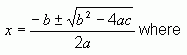SEARCH HOMEMath Central Quandaries & QueriesQuestion from amy, a parent: My son was given the following question:  The path of a large arrow fired from a non torsion catapult can be modeled by y=-0.0044x^2 + 1.68x, where x is the distance the arrow travelled (in yards) and y is the height of the arrow (in yards).  Given the height of a castle wall, find the safest distance from the wall to launch an arrow over the wall. The given height of the castle wall is 120yards. We are stumped by this.  I have started with 120=-0.0044x^2 + 1.68x But that does not compute to a regular x value. I have asked multiple math types who have given me this same formula.  I can only assume that we and they are not understanding how to do this. Any help would be most appreciated!Amy, we have two responses for you:

Hi Amy,

I'd try finding the maximum value of the height of the arrow (that is the maximum value of y -- you can use calculus or recognize that the function given is a parabola). If my quick calculation is right, the arrow reaches a maximum height of a little over 160 yards.

The value of x at which this occurs would be the distance away from the wall to fire the arrow from so that it reaches the top of its arc just as it crosses the wall.

I don't know if this is the "safest" distance to fire from, but I would guess that is what is being asked.

I hope this helps.

Victoria

Hi Amy.

I'm not sure why you say that these don't give a "regular x value": in fact you will get two x values corresponding to where the arrow is 120 yards up. One value (the smaller one) is where the arrow is still ascending and the other is where the arrow is descending. I would guess that the safest distance to launch your arrow from is the furthest from the wall in which the arrow can still cross over the wall.

Re-arrange your equation to make it equal zero:

0.0044x2 - 1.68x + 120 = 0

And use the Quadratic Formula to find the two values of x:a = 0.0044, b = -1.68, c = 120

I get a sensible answer. Give your calculator another attempt.

Cheers,
Stephen La Rocque.

PS: A castle wall that is 120 yards high?!? Wow!Math Central is supported by the University of Regina and The Pacific Institute for the Mathematical Sciences.